Effective ICSE S Chand Maths Class 9 Solutions Chapter 19 Trigonometrical Ratios Ex 19(C) can help bridge the gap between theory and application.

## S Chand Class 9 ICSE Maths Solutions Chapter 19 Trigonometrical Ratios Ex 19(C)

Question 1.
(i) Prove that sin 60° = 2 sin 30° cos 30°.
(ii) Without using trigonometric tables find the value of 3 sin2 45° + 2 cos2 60°.
Solution:
(i) L.H.S. = sin 60° = $$\frac{\sqrt{3}}{2}$$
R.H.S. = 2 sin 30° cos 30°
IMG
= 2 × $$\frac { 1 }{ 2 }$$ × $$\frac{\sqrt{3}}{2}$$ = $$\frac{\sqrt{3}}{2}$$
Hence sin 60° = 2 sin 30° cos 30°

(ii) 3 sin2 45° + 2 cos2 60°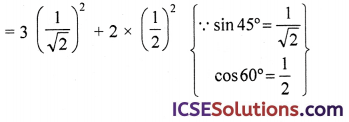= $$\frac { 3 }{ 2 }$$ + 2 × $$\frac { 1 }{ 4 }$$
= $$\frac { 3 }{ 2 }$$ + $$\frac { 1 }{ 2 }$$ = $$\frac{3+1}{2}$$ = $$\frac { 4 }{ 2 }$$ = 2

Question 2.
If 0 ≤ x ≤ 90°, state the numerical value of x for which sin x° = cos x°.
Solution:
sin x° = cos x° ⇒ $$\frac{\sin x}{\cos x}$$ = 1
⇒ tan x = 1 = tan 45° {∵ tan 45° = 1}
comparing, we get
x = 45°

Question 3.
Find the value of :
(i) sin2 60° + cos° 45°
(ii) 3 cos° 30° + tan2 60°
(iii) 4 sin2 60° + 3 tan2 30° – 8 sin 45° cos 45°
(iv) 2 sin2 30° – 3 cos2 45° + tan2 60°
(v) $$\frac{\sin 60^{\circ}}{\cos ^2 45^{\circ}}$$ – 3 tan 30° + 5 cos 90°
(vi) cos 90° + cos2 45° sin 30° tan 45°
(vii) cos2 45° + sin2 60° + sin2 30°
(viii) sin2 30° + cos2 60°
(ix) $$\frac{\sin ^2 45^{\circ}+\cos ^2 45^{\circ}}{\tan ^2 60^{\circ}}$$
(x) $$\frac{5 \sin ^2 30^{\circ}+\cos ^2 45^{\circ}-4 \tan ^2 30^{\circ}}{2 \sin 30^{\circ} \cos 30^{\circ}+\tan 45^{\circ}}$$
(xi) 2√2 cos 45°. cos 60° + 2√3 sin 30°. tan 60° – cos 0°
(xii) $$\frac { 4 }{ 3 }$$ tan2 30° + sin2 60° – 3 cos2 60° + $$\frac { 3 }{ 4 }$$ tan2 60° – 2 tan2 45°
(xiii) (cos 0° + sin 45° + sin 30°) × (sin 90° – cos 45° + cos 60°)
Solution: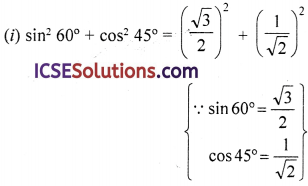= $$\frac { 3 }{ 4 }$$ + $$\frac { 1 }{ 2 }$$
= $$\frac{3+2}{4}$$ = $$\frac{5}{4}$$ = $$1 \frac{1}{4}$$

(ii) 3 cos2 30° + tan2 60° = 3 $$\left(\frac{\sqrt{3}}{2}\right)^2$$ + $$(\sqrt{3})^2$$
= 3 × $$\frac { 3 }{ 4 }$$ + 3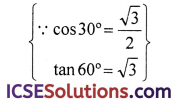= $$\frac { 9 }{ 4 }$$ + 3 = $$\frac{9+12}{4}$$ = $$\frac{21}{4}$$ = $$5 \frac{1}{4}$$

(iii) 4 sin2 60° + 3 tan2 30° – 8 sin 45° cos 45°(iv) 2 sin2 30° – 3 cos2 45° + tan2 60°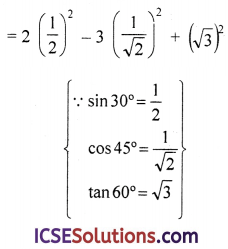= 2 × $$\frac{1}{4}$$ – 3 × $$\frac{1}{2}$$ + 3
= $$\frac{1}{2}$$ – $$\frac{3}{2}$$ + 3
= $$\frac{1-3+6}{2}$$ = $$\frac{4}{2}$$ = 2

(v) $$\frac{\sin 60^{\circ}}{\cos ^2 45^{\circ}}-3 \tan 30^{\circ}+5 \cos 90^{\circ}$$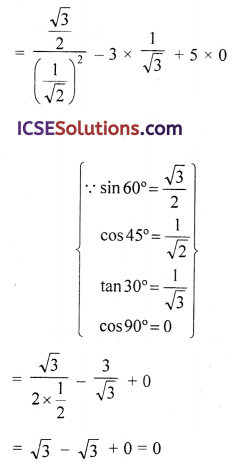(vi) cos 90° + cos2 45° sin 30° tan 45°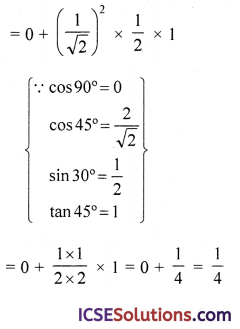(vii) cos2 45° + sin2 60° + sin2 30°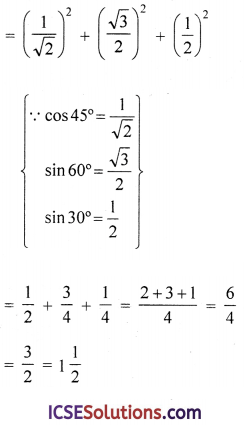(viii) sin2 30° + cos2 60°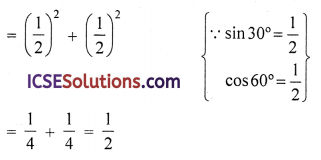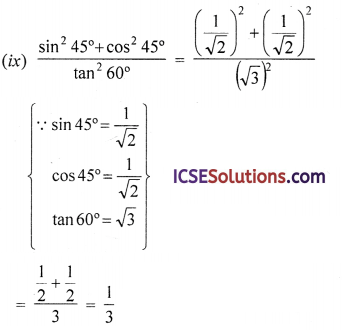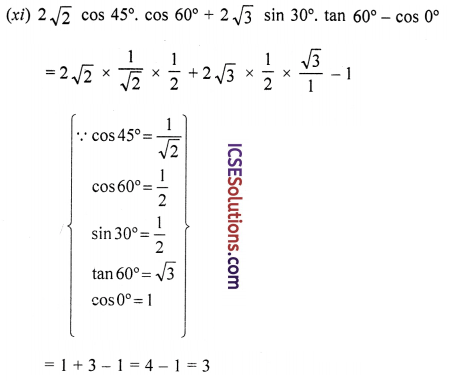(xii) $$\frac{4}{3}$$ tan2 30° + sin2 60° – 3 cos2 60° + $$\frac{3}{4}$$ tan2 60° – 2 tan 45°
= $$\frac{4}{3}$$ $$\left(\frac{1}{\sqrt{3}}\right)^2$$ + $$\left(\frac{\sqrt{3}}{2}\right)^2$$ – 3 $$\left(\frac{1}{2}\right)^2$$ + $$\frac{3}{4}$$ (√3)2 – 2(1)2
= $$\frac{4}{3}$$ × $$\frac{1}{3}$$ + $$\frac{3}{4}$$ – 3 × $$\frac{1}{4}$$ + $$\frac{3}{4}$$ x 3 – 2
= $$\frac{4}{9}$$ + $$\frac{3}{4}$$ – $$\frac{3}{4}$$ + $$\frac{9}{4}$$ – $$\frac{2}{1}$$
= $$\frac{16+27-27+81-72}{36}$$ = $$\frac{25}{36}$$

(xiii) (cos 0° + sin 45° + sin 30°) × (sin 90° – cos 45° + cos 60°)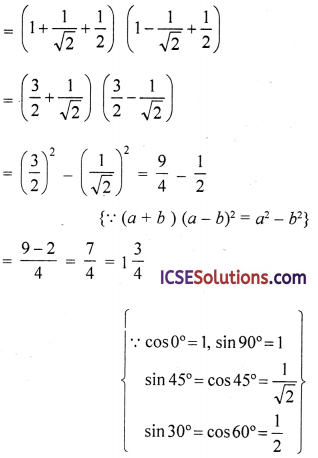Question 4.
ABC is an isosceles right triangle. Assuming AB = BC = x, find the value of each of the following trigonometric ratios.
(i) sin 45°
(ii) cos 45°
(iii) tan 45°
Solution:
In △ABC, AB = AC = x
Draw AD ⊥ BC which bisects BC at D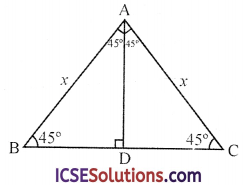∵ ∠B = 45°, then ∠C = 45°
and ∠A = 90°
( ∵AD is the perpendicular which bisect ∠A)
∴ BD = DC = AD = $$\frac { 1 }{ 2 }$$BC
∵ ∠A = 90°
∴ BC2 = AB2 + AC2
= x2 + x2 = 2x2
BC = √2x
∴ BD = DC = AD = $$\frac{\sqrt{2} x}{2}$$
Now (i) sin 45° = $$\frac{\text { AD }}{\text { AB }}$$ = $$\frac{\frac{\sqrt{2 x}}{2}}{x}$$
= $$\frac{\sqrt{2}}{2}$$ = $$\frac{1}{\sqrt{2}}$$
cos 45° = $$\frac{\text { BD }}{\text { AB }}$$ = $$\frac{\frac{\sqrt{2} x}{2}}{x}$$ = $$\frac{\sqrt{2}}{2}$$ = $$\frac{1}{\sqrt{2}}$$
tan 45° = $$\frac{\text { AD }}{\text { BD }}$$ = $$\frac{\frac{\sqrt{2}}{2} x}{\frac{\sqrt{2}}{2} x}$$ = 1

Question 5.
Without using tables, find the value of
$$\frac{\sin 30^{\circ}-\sin 90^{\circ}+2 \cos 0^{\circ}}{\tan 30^{\circ} \times \tan 60^{\circ}}$$
Solution:
$$\frac{\sin 30^{\circ}-\sin 90^{\circ}+2 \cos 0^{\circ}}{\tan 30^{\circ} \times \tan 60^{\circ}}$$ = $$\frac{\frac{1}{2}-1+2 \times 1}{\frac{1}{\sqrt{3}} \times \sqrt{3}}$$
= $$\frac{\frac{1}{2}-1+2}{1}$$ = $$\frac{1}{2}$$ – 1 + 2 = $$1 \frac{1}{2}$$ = 1.5

Question 6.
Without using tables verify that :
(i) sin 60° = $$\frac{2 \tan 30^{\circ}}{1+\tan ^2 30^{\circ}}$$ = $$\frac{\sqrt{3}}{2}$$;
(ii) cos 60° = $$\frac{1-\tan ^2 30^{\circ}}{1+\tan ^2 30^{\circ}}$$ = $$\frac{1}{2}$$;
(iii) cos 60° = cos2 30° – sin2 30°
(iv) cos 60° = 1 – 2 sin2 30° = 2 cos2 30° – 1.
Solution:
(i) sin 60° = $$\frac{2 \tan 30^{\circ}}{1+\tan ^2 30^{\circ}}$$ = $$\frac{\sqrt{3}}{2}$$
sin 60° = $$\frac{\sqrt{3}}{2}$$
and $$\frac{2 \tan 30^{\circ}}{1+\tan ^2 30^{\circ}}$$ = $$\frac{2 \times \frac{1}{\sqrt{3}}}{1+\left(\frac{1}{\sqrt{3}}\right)^2}$$ = $$\frac{\frac{2}{\sqrt{3}}}{1+\frac{1}{3}}$$
= $$\frac{\frac{2}{\sqrt{3}}}{\frac{4}{3}}$$ = $$\frac{2}{\sqrt{3}}$$ × $$\frac{3}{4}$$ = $$\frac{\sqrt{3}}{2}$$
Hence sin 60° = $$\frac{2 \tan 30^{\circ}}{1+\tan ^2 30^{\circ}}$$ = $$\frac{\sqrt{3}}{2}$$

(ii) cos 60° = $$\frac{1-\tan ^2 30^{\circ}}{1+\tan ^2 30^{\circ}}$$ = $$\frac{1}{2}$$
cos 60° = $$\frac{1}{2}$$
and $$\frac{1-\tan ^2 30^{\circ}}{1+\tan ^2 30^{\circ}}$$ = $$\frac{1-\left(\frac{1}{\sqrt{3}}\right)^2}{1+\left(\frac{1}{\sqrt{3}}\right)^2}$$ = $$\frac{1-\frac{1}{3}}{1+\frac{1}{3}}$$
= $$\frac{\frac{2}{3}}{\frac{4}{3}}$$ = $$\frac{2}{3}$$ × $$\frac{3}{4}$$ = $$\frac{1}{2}$$
Hence cos 60° = $$\frac{1-\tan ^2 30^{\circ}}{1+\tan ^2 30^{\circ}}$$ = $$\frac{1}{2}$$

(iii) cos 60° = cos2 30° – sin2 30°
cos 60° = $$\frac{1}{2}$$
and cos2 30° – sin2 30° = $$\left(\frac{\sqrt{3}}{2}\right)^2$$ – $$\left(\frac{1}{2}\right)^2$$
= $$\frac{3}{4}$$ – $$\frac{1}{4}$$
= $$\frac{2}{4}$$ = $$\frac{1}{2}$$
Hence cos 60° = cos2 30° – sin2 30°

(iv) cos 60° = 1 – 2sin2 30° = 2 cos2 30° – 1
cos 60° = $$\frac{1}{2}$$
1 – 2sin2 30° = 1 – 2 × $$\left(\frac{1}{2}\right)^2$$ = 1 – 2 × $$\frac{1}{4}$$
= 1 – $$\frac{1}{2}$$ = $$\frac{1}{2}$$
and 2 cos2 30° – 1 = 2 $$\left(\frac{\sqrt{3}}{2}\right)^2$$ – 1
= 2 × $$\frac{3}{4}$$ – 1
= $$\frac{3}{4}$$ – 1 = $$\frac{1}{2}$$
Hence cos 60° = 1 – 2 sin2 30°
= 2 cos2 30° – 1Question 7.
Prove that:
(i) cos2 30° + sin2 30° + tan2 45° = 2.
(ii) 4 (sin4 30° + cos4 60°) – 3 ( cos2 45° – sin2 90°) = 2.
Solution:
(i) L.H.S. = cos2 30° + sin2 30° + tan2 45°
= $$\left(\frac{\sqrt{3}}{2}\right)^2$$ + $$\left(\frac{1}{2}\right)^2$$ + (1)2
= $$\frac{3}{4}$$ + $$\frac{1}{4}$$ + 1 = 1 + 1 = 2 = R.H.S.

(ii) L.H.S. = 4 (sin 4 30° 1+ cos 4 60°) – 3 (cos2 45° – sin2 90°)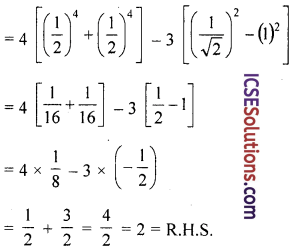Question 8.
A pole 15 m long rests against a vertical wall at an angle of 60° with the ground. Calculate
(i) how high up the wall will the pole reach?
(ii) how far is the foot of the pole from the wall?
Solution:
AC is wall and AB is a pole which rests with wall at 60°
In right △ABC, ∠C = 90°
AB = 15 m
Let height of wall = x m
and away from foot of wall = y m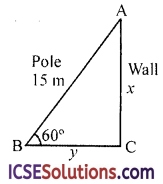(i) ∵ sin 60° = $$\frac{\text { AC }}{\text { AB }}$$
⇒ $$\frac{\sqrt{3}}{2}$$ = $$\frac{x}{15}$$ ⇒ x = $$\frac{15 \sqrt{3}}{2}$$
⇒ x = $$\frac{15(1.732)}{2}$$ = $$\frac{25.980}{2}$$ = 12.99 m
∴ Height of wall = 12.99 m

(ii) cos 60° = $$\frac{\text { BC }}{\text { AB }}$$ ⇒ $$\frac{1}{2}$$ = $$\frac{y}{15}$$
⇒ y = $$\frac{15}{2}$$ = 7.5 m
∴ Distance from the foot of wall = 7.5 m

Question 9.
In the given triangle, calculate θ if B = 90°, AB = 20 cm and AC = 40 cm.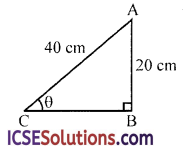Solution:
In the △ABC, ∠B = 90° and
∠C = θ, AC = 40 cm, AB = 20 cm
Here sin θ = $$\frac{\text { AB }}{\text { AC }}$$ = $$\frac{20}{40}$$ = $$\frac{1}{2}$$
= sin 30° {∵ sin 30° = $$\frac{1}{2}$$}
∴ θ = 30°

Question 10.
Without using tables solve each of the following triangle ABC, right-angled at C; given
(i) A = 30° and c = 40;
(ii) B = 60° and c = 15;
(iii) A = 45° and a = 7.
Solution:(i) In △ABC, ∠c = 90°
∠A = 30 and c = 40
sin θ = $$\frac{\text { BC }}{\text { AB }}$$ = $$\frac{a}{c}$$
⇒ sin 30° = $$\frac{a}{c}$$ ⇒ $$\frac{1}{2}$$ = $$\frac{a}{40}$$
⇒ a = $$\frac{40}{2}$$ = 20
But c2 = a2 + b2 (Pythagoras Theorem)
(40)2 = (20)2 +b2 ⇒ 1600 – 400 = 1200
= 400 × 3
∴ b = $$\sqrt{400 \times 3}$$ = 20√3 = 20(1.732)
= 34.64 = 34.64
a = 20 and b = 34.64 and
∠B = 90° – 30° = 60°

(ii) ∠B = 60° and c = 15
sin θ = $$\frac{\text { AC }}{\text { AB }}$$ ⇒ sin 60° = $$\frac{a}{c}$$
⇒ $$\frac{\sqrt{3}}{2}$$ = $$\frac{a}{15}$$ ⇒ a = $$\frac{15 \sqrt{3}}{2}$$
⇒ a = $$\frac{15(1.732)}{2}$$ = 12.99
We know that by Pythagoras Theorem,
(15)2 = $$\left(\frac{15 \sqrt{3}}{2}\right)^2$$ + b2 ⇒ 225 = $$\frac{675}{4}$$ + b2
⇒ b2 = 225 – $$\frac{675}{4}$$
= $$\frac{900-675}{4}$$ = $$\frac{225}{4}$$
∴ b = $$\sqrt{\frac{225}{4}}$$ = $$\frac{15}{2}$$ = 7.5
and ∠A = 90° – B = 90° – 60° = 30°

(iii) A = 45° and a = 7
sin θ = $$\frac{\text { BC }}{\text { AC }}$$ ⇒ sin 45° = $$\frac{a}{c}$$
⇒ $$\frac{1}{\sqrt{2}}$$ = $$\frac{7}{c}$$ ⇒ c = $$7 \sqrt{2}$$ = 7(1.414)
⇒ c = 9.898 = 9.9
But C2 = a2 + b2
⇒ (7√2)2 = (7)2 + b2 ⇒ 98 = 49 + b2
⇒ b2 = 98 – 49 = 49 = (7)2
∴ b = 7
Hence c = 9.9 and b = 7
and ∠B = 90° – ∠A = 90° – 45° = 45°Question 11.
If 4 sin2 θ – 1 = 0 and angle θ is less than 90°; find the value of θ and hence the value of cos2 θ + tan2 θ.
Solution:
4 sin2 θ – 1 = 0 ⇒ 4 sin 2 θ = 1
⇒ sin 2 θ = $$\frac{1}{4}$$ = $$\left(\frac{1}{2}\right)^2$$

(i) ∴ sin θ = $$\frac{1}{2}$$ = sin 30° {∵ sin 30° = $$\frac{1}{2}$$}
∴ θ = 30°
Now cos2 θ + tan2 θ = cos2 30° + tan2 30°
= $$\left(\frac{\sqrt{3}}{2}\right)^2$$ + $$\left(\frac{1}{\sqrt{3}}\right)^2$$
= $$\frac{3}{4}$$ + $$\frac{1}{3}$$
= $$\frac{9+4}{12}$$ = $$\frac{13}{1}$$ = $$1 \frac{1}{12}$$

Question 12.
If θ is an acute angle and sin θ = cos θ, find the value of 2 tan2 θ + sin2 θ – 1.
Solution:
(i) ∵ sin θ = cos θ ⇒ $$\frac{\sin \theta}{\cos \theta}$$ = 1
⇒ tan θ = 1 = tan 45° {∵ tan 45° = 1}
∴ θ = 45°

(ii) Now 2 tan2 θ + sin2 θ – 1
=2 tan2 45° + sin2 45° – 1= 2 (1)2 + $$\left(\frac{1}{\sqrt{2}}\right)^2$$ – 1
= 2 × 1 + $$\frac{1}{2}$$ – 1 = 2 + $$\frac{1}{2}$$ – 1
= $$1 \frac{1}{2}$$ = $$\frac{3}{2}$$ = 1.5

Question 13.
A kite, flying at a height of 75 metres from the level ground, is attached to a string inclined at 60° to the horizontal, find the length of the string to the nearest metre.
Solution:
Height of the kite = 75 m
Angle of elevation = 60°∴ JK is the string tied to the kite
Let JK = x m
Now sin θ = $$\frac{\text { KL }}{\text { JK }}$$
⇒ sin 60° = $$\frac{75}{x}$$ ⇒ $$\frac{\sqrt{3}}{2}$$ = $$\frac{75}{x}$$
⇒ x = $$\frac{75 \times 2}{\sqrt{3}}$$ = $$\frac{150 \times \sqrt{3}}{\sqrt{3} \times \sqrt{3}}$$ m
= $$\frac{150 \sqrt{3}}{3}$$ = 50√3 = 50(1.732)
= 86.600 m = 87 m

Question 14.
If 2 sin θ – 1 = 0, find :
(i) the value of θ in degrees where θ is an acute angle;
(ii) cos2θ + tan2θ.
Solution:
(i) 2 sin θ – 1 = 0 ⇒ 2 sin θ = 1
⇒ sin θ = $$\frac{1}{2}$$ = sin 30° {∵ sin 30° = $$\frac{1}{2}$$}
∴ θ = 30°

(ii) Now, cos2θ + tan2θ = cos2 30° + tan2 30°
= $$\left(\frac{\sqrt{3}}{2}\right)^2$$ + $$\left(\frac{1}{\sqrt{3}}\right)^2$$ = $$\frac{3}{4}$$ + $$\frac{1}{3}$$
= $$\frac{9+4}{12}$$ = $$\frac{13}{12}$$ = $$1 \frac{1}{12}$$

Question 15.
The altitude AD of a △ABC, in which ∠A is obtuse, is 10 cm. If BD = 10 cm and CD = 10√3 cm, determine ∠A.
Solution:
In △ABC, ∠A is an obtused angle AD ⊥ BC,
BD = 10 cm and CD = 10√3 cm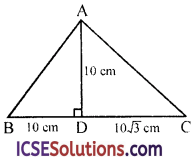∠A = α + β
Now tan α = $$\frac{\text { BD }}{\text { AD }}$$ = $$\frac{10}{10}$$ = 1 = tan 45° {∵ tan 45° = 1}
∴ α =45°
and tan β = $$\frac{\text { CD }}{\text { AD }}$$ = $$\frac{10 \sqrt{3}}{10}$$ = √3 = tan 60° {∵ tan 60° = √3}
∴ β = 60°
Now ∠A = α + β = 45° + 60° = 105°

Question 16.
In a right triangle ABC, right angled at C, if ∠B = 60° and AB = 15 units, find the remaining angles and sides.
Solution:
In △ABC, ∠C = 90°, ∠B = 60°,
AB = 15 units∴ ∠A = 180° – (90° + 60°)
= 180° – 150° = 30°
Now sin θ = $$\frac{\text { AC }}{\text { AB }}$$ ⇒ sin 60° = $$\frac{\text { AC }}{\text { AB }}$$
⇒ $$\frac{\sqrt{3}}{2}$$ = $$\frac{\mathrm{AC}}{15}$$ ⇒ AC = $$\frac{15 \sqrt{3}}{2}$$ = $$\frac{15}{2}$$ √3 units
and cos 60° = $$\frac{\text { BC }}{\text { AB }}$$ ⇒ $$\frac{1}{2}$$ = $$\frac{\mathrm{BC}}{15}$$
⇒ BC = $$\frac{15}{2}$$ = 7.5 units
Hence ∠B = 30°, BC = 7.5 units, AC = $$\frac{15}{2}$$√3 units

Question 17.
In a rectangle ABCD, AB = 20 cm, ∠BAC = 60°, calculate side BC and diagonals AC and BD.
Solution:
In reactangle ABCD, AB = 20 cm
∠BAC = 60° and AC and BD are its two diagonals which are equal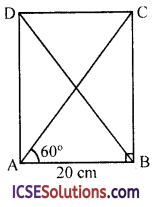tan ∠BAC = $$\frac{\text { BC }}{\text { AB }}$$
⇒ tan 60° = $$\frac{\mathrm{BC}}{20}$$ ⇒ √3 = $$\frac{\mathrm{BC}}{20}$$
⇒ BC = 20√3 cm
Similarly cos 60° = $$\frac{\text { AB }}{\text { AC }}$$ = $$\frac{20}{A C}$$
⇒ $$\frac{1}{2}$$ = $$\frac{20}{A C}$$ = AC = 20 × 2 = 40 cm
∵ BD = AC
⇒ BD = 40 cmQuestion 18.
A ladder is placed against a wall such that it just reaches the top of the wall. The foot of the ladder is 1.5 m away from the wall and the ladder is inclined at an angle of 60° with the ground. Find the height of the wall. (Given √3 = 1.73)
Solution:
Let AB is wall and AC is ladder which is placed against the wall and makes an angle of 60° with the ground
Foot of the ladder is 1.5 m away from the wall i.e. BC = 1.5 mLet h be the height of the wall then
tan C = $$\frac{\text { AB }}{\text { AC }}$$ ⇒ tan 60° = $$\frac{h}{1.5}$$
⇒ √3 = $$\frac{h}{1.5}$$ ⇒ h = 1.5√3
⇒ h = (1.5) (1.73) m = 2.595 m
∴ Height of the wall = 2.595 m = 2.59 m

Question 19.
An electric pole is 10 m high. A steel wire tied to the top of the pole is affixed at a point on the ground to keep the pole upright. If the steel wire makes an angle 45° with the horizontal through the foot of the pole, find the length of the steel wire. (Given √2 = 1.41)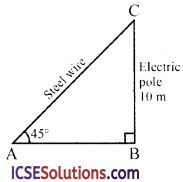Solution:
BC is the electric pole and AC is the wire tied to it such that
∠A = 45° and BC = 10 m Let AC = x
Now sin A = $$\frac{\text { BC }}{\text { AC }}$$
⇒ sin 45° = $$\frac{10}{x}$$
⇒ $$\frac{1}{\sqrt{2}}$$ = $$\frac{10}{x}$$
⇒ x = 10√2 = 10 (1.414) m = 14.14 m
Length of wire = 14.14 = 14.1 m

Question 20.
A vertically straight tree, 15 m high, is broken by the wind in such a way that its top just touches the ground and makes an angle of 60° with the ground. At what height from the ground did the tree break ? (Use √3 = 1.73)Solution:
Let DB is a tree which is broken from A and makes an angle of 60° with the ground then AD = AC and DB = 15 cmLet AB = x, then
AC = AD = (15 – x) m
Now, sin θ = $$\frac{\text { Perp. }}{\text { Hyp. }}$$ ⇒ sin 60° = $$\frac{\text { AB }}{\text { AC }}$$
⇒ $$\frac{\sqrt{3}}{2}$$ = $$\frac{x}{15-x}$$ ⇒ 2x = 15√3 = √3 x
⇒ 2x + √3 x = 15√3
⇒ (2 + √3) x = 15√3
∴ x = $$\frac{15 \sqrt{3}}{2+\sqrt{3}}$$ = $$\frac{15 \sqrt{3}(2-\sqrt{3})}{(2+\sqrt{3})(2-\sqrt{3})}$$
= $$\frac{30 \sqrt{3}-45}{4-3}$$
= $$\frac{30(1.73)-45}{1}$$ = 51.9 – 45 = 6.9 m
∴ Height of tree from which it was broken = 6.9 m

Question 21.
The string of a kite is 150 m long and it makes an angle of 60° with the horizontal. Find the height of the kite from the ground. (Take √3 = 1.73).
Solution:
K is kite and KL is string tied to it which makes an angle of 60° with the ground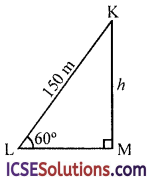∴ ∠L = 60°, LK = 150 m
Let KM = h m
sin θ = $$\frac{\text { Perp. }}{\text { Hyp. }}$$
⇒ sin 60° = $$\frac{\text { KM }}{\text { KL }}$$
⇒ $$\frac{\sqrt{3}}{2}$$ = $$\frac{h}{150}$$ ⇒ h = $$\frac{150 \sqrt{3}}{2}$$ = 75√3 m
∴ Height of teh kite = 75√3 = 75 x 1.73 m = 129.75 = 129.8 m